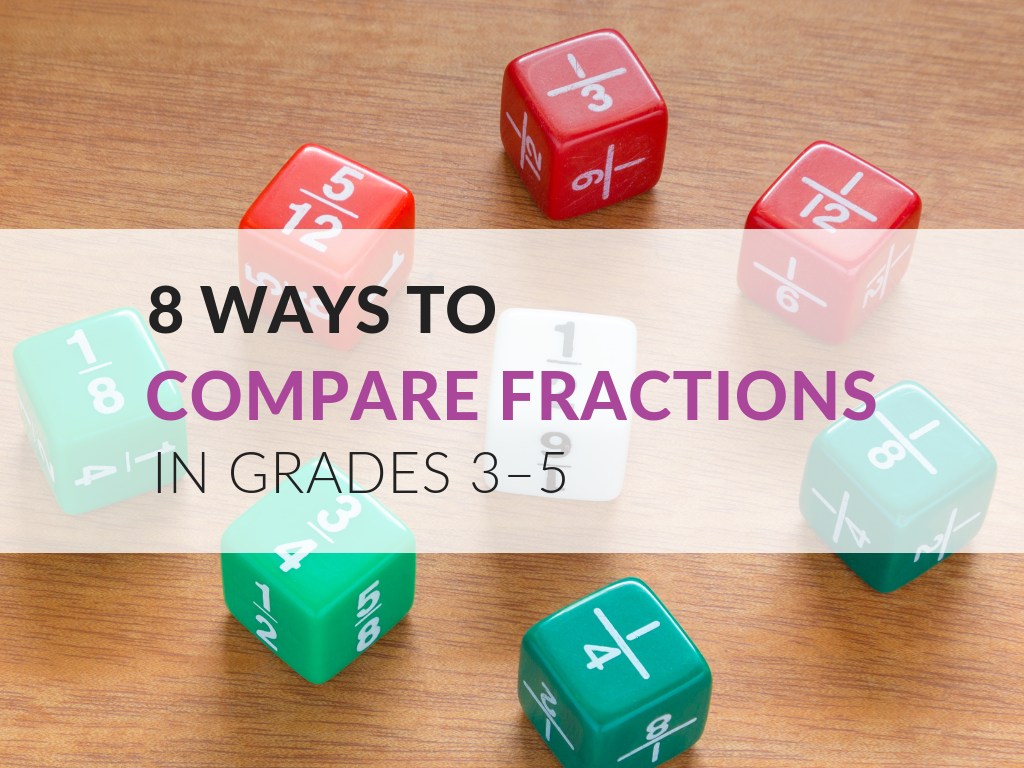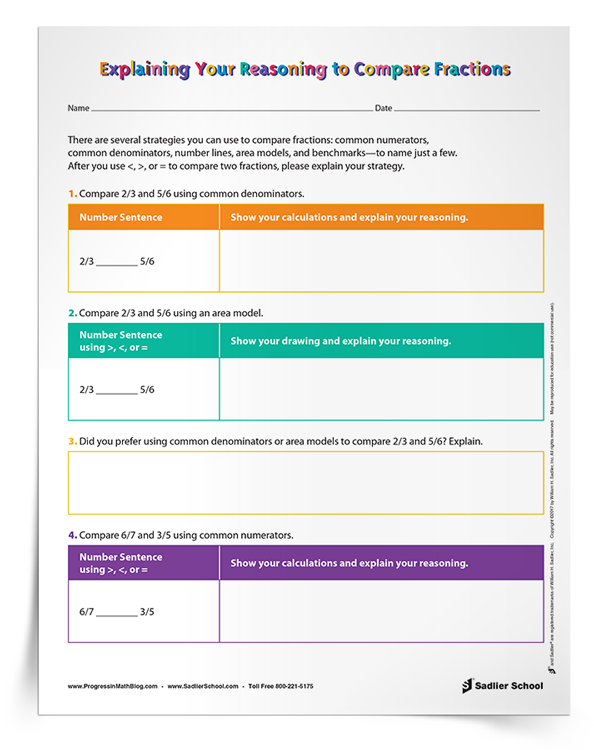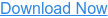1.800.221.5175
Core Program
Full Access
Core Program
Full Access
High-Impact Tutoring
Core Program
Full Access
National Program
Full Access
New York
Critical Thinking for Active Math Minds
Preparing for Standards Based Assessments
Vocabulary Workshop, Tools for Comprehension Grades 1–5
Print Program
Interactive Edition
Print Program
Interactive Edition
Vocabulary Workshop, Tools for Excellence Grades 6–12+
Print Program
Interactive Edition
Vocabulary for Success
Progress English Language Arts Grades K–8
National Program
Full Access
High-Impact Tutoring
New York
Grammar Workshop, Tools for Writing
Grammar for Writing
Print Program
Interactive Practice Bundle
Fluency Booster Practice Book
Interactive Assessments
High-Impact Tutoring
Building Reading Success with Wiley Blevins
Print Program
Interactive Edition
Professional Learning

A K–8 resource to support deep comprehension of math skills and conceptsTopics:

#### March 15, 2023 3-5-number-and-operations-fractions

How do you compare fractions in the late elementary grades? In this post, I want to share a collection of methods that you can use with students, in Grades 3–5, to compare fractions. Plus, download a FREE set of problems in which students are asked to use and think about different strategies for comparing fractions.When I was younger, I only learned one way to compare fractions. It wasn’t until I was tutoring math as a community college student that I started to explore the different ways of representing and comparing fractions. There have been a few times as a teacher when I have had “Aha!” moments that have given me even deeper insights into how to compare fractions.

Students’ ability to justify their choice of strategy for comparing fractions is part of Standard for Mathematical Practice 3, “construct viable arguments and critique the reasoning of others.”

# How to Compare Fractions In Grades 3–5

Below I've outlined eight ways to compare fractions. Once you develop these different strategies for how to compare fractions with your students, check their reasoning skills using the download for this post.

My printable Explaining Your Reasoning to Compare Fractions Activity asks students to compare fractions using given strategies, by choosing between strategies, and by creating a problem of their own.## 1. Equivalent Denominators

This is the easiest situation in which to compare fractions. If two fractions have equivalent denominators, then compare the numerators to determine which faction is greater. Students at the earliest stages of learning about fractions should be able to do this.

## 2. Equivalent Numerators

I was quite surprised to learn about this a few years ago. If two fractions have equivalent numerators, then the fraction with the lesser denominator has a greater value. The first time I heard this strategy, I am pretty sure that I disagreed with the person who told me it was possible! It is based on the idea that unit fractions with lesser denominators have a greater value than unit fractions with greater denominators (for instance, ⅓ is smaller than ½). This concept can be generalized to any fractions with common numerators—but I had to be convinced of this, as I had never learned it in grade school.

## 3. Related Denominators

If two fractions have denominators that are easily related (such as 4 and 8), then it is easy to scale one of them up quickly in order to compare them. This is particularly useful when working with ruler measurement and line plots using halves, fourths, and eighths. Using measurement and data topics in Grades 3 to 5 is an important way to support students’ reasoning about comparing fractions with denominators 2, 4, and 8.

## 4. Related Numerators

If two fractions have numerators that are easily related (such as 3 and 6), then scaling the fraction with the lesser numerator to be equivalent to the greater numerator allows comparison based on their denominators. For instance, to compare 3/4 and 6/7, scale 3/4 to be 6/8. Comparing 6/8 and 6/7 is easy if one knows that 1/7 is greater than 1/8.

## 5. Number Line Models

Subdividing a unit length on a number line in order to compare two fractions is a great way to assess students’ comprehension of fractions. I like to let students use whiteboards for constructions like these, as they can more accurately refine their subdivisions if needed.

## 6. Area Models

Circles, squares, and rectangles are popular choices for subdividing in order to compare fractions. Not only can the teacher assess students’ equal subdivisions, but also the idea of equally-sized unit wholes.

## 7. Benchmarks

Sometimes comparing fractions to benchmarks such as 0, ½, or 1 is a quick way to compare fractions. For instance, when comparing 5/9 to 6/13, students can explain their reasoning by stating that 5/9 is more than ½ while 6/13 is less than ½.

## 8. Cross Multiplication

While I have had many a debate with teachers about whether to use this method to compare fractions, it must be included on the list. If the product of the first numerator and the second denominator is greater than the product of the second numerator and the first denominator, then the first fraction is greater than the second.

In Summary

As you can see, students have many options when comparing fractions. There are even more ways (converting to decimals, for instance) that will become important in later grades.

Hopefully, the information and printable download featured in this post will help you build students’ ability to justify their choice of strategy for comparing fractions!